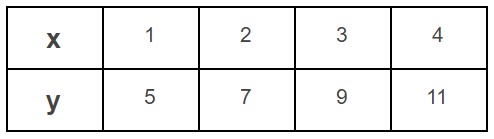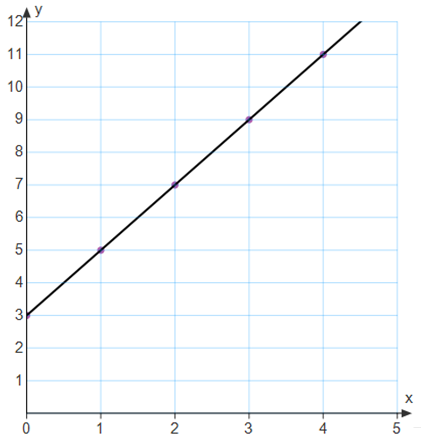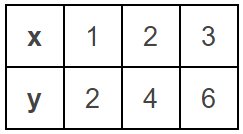• If you are citizen of an European Union member nation, you may not use this service unless you are at least 16 years old.

View

# 6-A-1-2

last edited by 2 years, 2 months ago

6.A.1.2 Represent relationships between two varying quantities involving no more than two operations with rules, graphs, and tables; translate between any two of these representations.

In a Nutshell

Identifying patterns in math can be done by determining the relationship (finding the rule) between consecutive numbers in a sequence, by examining the changes in ordered pairs on a graph, or by identifying the changes between input and output values in a table. While the patterns may be simple, such as “adding one” or “multiplying by 3,” more complex patterns may emerge which involve two operations such as first multiplying, and then adding. Examination of at least three numbers (sequence), ordered pairs (graph), or entries (table) must be completed to determine the pattern.

## Teacher Actions

• Develop strategies for problem solving as students engage in tasks that provide opportunities for them to recognize, explore, and discover patterns associated with a sequence, graph, or table.

• Develop mathematical reasoning when finding patterns by identifying and applying the rule associated with a sequence, graph, or table.

• Develop the ability to model mathematical patterns using rules, graphs, or tables.

• Develop appropriate procedural fluency by translating between representations. (Ex: Translate data from an input/output chart to graphing on a coordinate grid.)

Rule:  2x + 3

Table:Graph:• Implement tasks that promote reasoning while students engage in discussion of problem solving strategies for translating between representations (rule, graph, table) while working with many different types of patterns.

• Pose purposeful questions to elicit student thinking about how the values being compared are related. (What evidence shows a relationship between two quantities?)

• Use and connect representations to engage students in exploring that the relationship between data may be represented in varied forms (numbers in a sequence, input/output charts/ tables, graphs, equations).

## Misconceptions

• Patterns are not discernable by only comparing two quantities, a third comparison must be made to validate the initial idea. For example, in the table below, the rule between the first input value and output value could be either “plus one” or “multiply by two.” Looking at the next two input values verifies that the rule is “multiply by two.”• Operations may be used together to create a pattern. (Ex: multiply by 2, then add 1)

• When translating between representations, the input value in a table may be represented as an x coordinate and the output value represented as a y coordinate on a graph.

• Believe that choosing any two quantities to compare will provide the rule.

• Incorrectly use additive or multiplicative relationships.

• Incorrectly graph values for input and output, placing the x and y coordinates in the wrong section of a graph.

• Incorrectly place the x and y coordinates in the wrong section of a table.

OKMath Framework Introduction